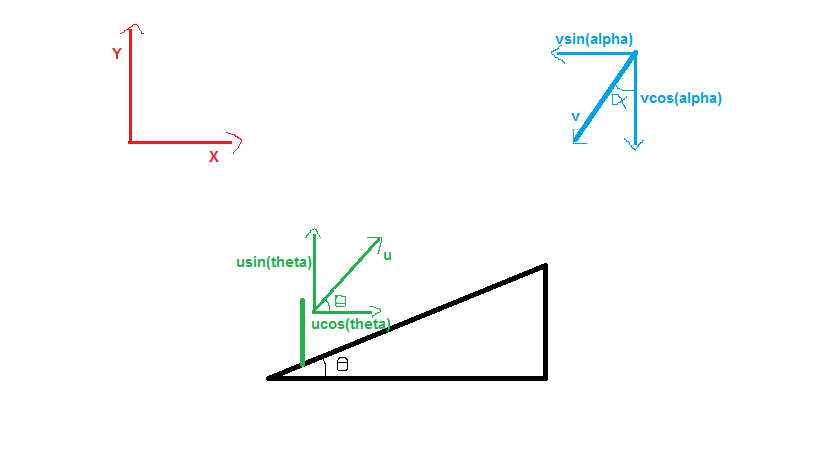# Help again

A man is running up an incline planr maaking an angle $\theta$ with horizontal with a speed u .Rain drops falling at an angle $\alpha$ with the vertical appear to the man as if they are falling at an angle of $45^o$ with the horizontal. The speed of rain drops isNote by Kyle Finch
5 years, 5 months ago

This discussion board is a place to discuss our Daily Challenges and the math and science related to those challenges. Explanations are more than just a solution — they should explain the steps and thinking strategies that you used to obtain the solution. Comments should further the discussion of math and science.

When posting on Brilliant:

• Use the emojis to react to an explanation, whether you're congratulating a job well done , or just really confused .
• Ask specific questions about the challenge or the steps in somebody's explanation. Well-posed questions can add a lot to the discussion, but posting "I don't understand!" doesn't help anyone.
• Try to contribute something new to the discussion, whether it is an extension, generalization or other idea related to the challenge.

MarkdownAppears as
*italics* or _italics_ italics
**bold** or __bold__ bold
- bulleted- list
• bulleted
• list
1. numbered2. list
1. numbered
2. list
Note: you must add a full line of space before and after lists for them to show up correctly
paragraph 1paragraph 2

paragraph 1

paragraph 2

[example link](https://brilliant.org)example link
> This is a quote
This is a quote
    # I indented these lines
# 4 spaces, and now they show
# up as a code block.

print "hello world"
# I indented these lines
# 4 spaces, and now they show
# up as a code block.

print "hello world"
MathAppears as
Remember to wrap math in $$ ... $$ or $ ... $ to ensure proper formatting.
2 \times 3 $2 \times 3$
2^{34} $2^{34}$
a_{i-1} $a_{i-1}$
\frac{2}{3} $\frac{2}{3}$
\sqrt{2} $\sqrt{2}$
\sum_{i=1}^3 $\sum_{i=1}^3$
\sin \theta $\sin \theta$
\boxed{123} $\boxed{123}$

Sort by:Take relative velocity along x and y axes and equate magnitude

- 5 years, 5 months ago

While talking about the rain, you took its direction in the south west direction. What prompted you to do that?? Why didn't you take it in the south-east direction?? In that case the x-component of relative velocity will be

ucos(theta)-vsin(alpha) and not ucos(theta)+vsin(alpha) as in this case...

- 5 years, 5 months ago

Both are equivalent. Just interchange $\alpha$ in my equation with $-\alpha$ to get to the result that you have mentioned. This disparity arises since I am measuring the angle in the clockwise direction, while you are measuring it in the anticlockwise direction.

- 5 years, 5 months ago

Yea, but the final expressions for the velocity are completely different...Then how are the results equivalent??

- 5 years, 5 months ago

Yes i solved it . Any way thanx for helping .

- 5 years, 5 months ago

$v=u \sin {(\pi/4 -\theta)} \csc {(\pi/4 -\alpha)}$

- 5 years, 5 months ago

- 5 years, 5 months ago

Can u explain in brief

- 5 years, 5 months ago